# Polyhedra - Puzzles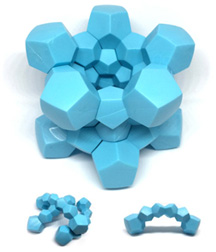Dodecaplex Puzzle \$24.95 Twelve regular 2D pentagons join together nicely in 3D to make a dodecahedron. 120 of those dodecahedra unite in 4D space to form the glorious hyper-dodecahedron! Dodecaplex is a physical puzzle made from sections of a 3D rendering of the hyper-dodecahedron - projected down into 3D, and split apart into pieces. The challenge is to put it back together, and there are three different solutions 5 or 6 of the pieces can be combined into. Includes color instructions and a storage pouch.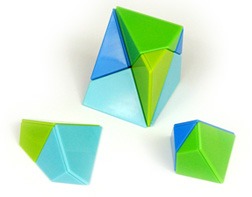Pentadron \$34.95 *** Not available at this time *** Pentadron consists of twelve plastic pieces with magnets inside that only allow them to fit together certain ways. The twelve pieces are all shaped the same, except for the fact that half are mirror images of the others. Assembling all twelve pieces into a cube is a challenging puzzle. This remarkable shape, a recent discovery by Jin Akiyama, is an elementary building block that allows construction of all five of Federov's parallel polyhedra. These space-filling polyhedra are the cube, the hexagonal prism, the rhombic dodecahedron, the elongated rhombic dodecahedron, and the truncated octahedron. (The four other than the cube take more than 12 pieces.) Ages 8 to adult.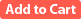The Irrational Puzzle \$19.95 This set contains four cubes dissected four different ways to form a variety of geometric solids. The instructions contain seven brain teasers in which different three-dimensional geometric shapes are formed with the pieces. This puzzle makes use of the fact that the face diagonal and space diagonal of a unit cube are the squares roots of 2 and 3, respectively, to introduce students to the concept of irrational numbers. Three reproducible worksheets cover the history of the square root of 2, the discovery of irrational numbers, and the Pythagorean Theorem. Contains 11 geometric solids made from soft, durable, and nontoxic foam. Cubes measure 2.5" on a side. Grades 3-12.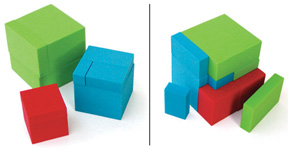Cubed Cubes \$14.95 Cubed Cubes is a brain teaser puzzle that teaches students about rectangular solids and the cubic numbers. The foam pieces form red, blue, and green cubes, each of a different size. In addition, all nine pieces together form a larger cube. The puzzle is based on the mathematical curiosity that the cubes of 3, 4 and 5 sum to the cube of 6. The instructions include several additional three-dimensional puzzles to solve using the same pieces. Also included are classroom worksheets on the volume of rectangular solids and cubic numbers. Grades 2-12.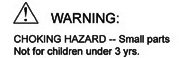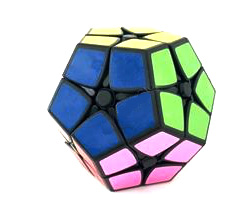Dodecahedron "2 x 2" Twisty Puzzle \$12.95 This "2 x 2" twisty puzzle is in the form of a regular dodecahedron.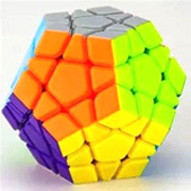Megaminx Dodecahedron Puzzle \$19.95 The 12 different colors of this high-quality rotational puzzle should throw you for a loop as to the solution. This version has 12 different colors of plastic rather than stickers. MoYu brand, 3 1/4 in x 3 1/4 in x 2 2/3 in.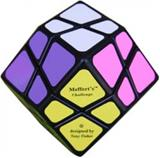Rhombic Dodecahedron Twisty Puzzle \$14.95 This twisty puzzle is in the form of a rhombic dodecahedron (twelve faces, each of which is a rhombus).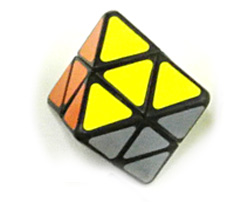Octahedron "2 x 2" Twisty Puzzle \$9.95 This "2 x 2" twisty puzzle is in the form of a regular octahedron (eight faces, each of which is an equilateral triangle).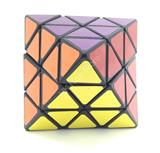Octahedron Twisty Puzzle \$14.95 This twisty puzzle is in the form of a regular octahedron (eight faces, each of which is an equilateral triangle).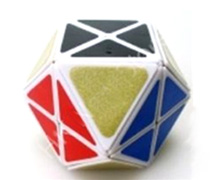Cuboctahedron Twisty Puzzle \$14.95 This "face-turning" twisty puzzle is in the form of a cuboctahedron (eight triangular faces and six square faces).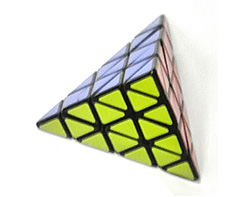Pyraminx 4x4 Tetrahedron Twisty Puzzle \$19.95 This "4 x 4" twisty puzzle is shaped like a regular tetrahedron, or triangular pyramid.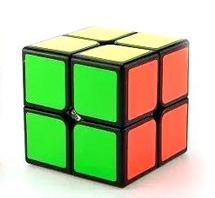2x2 Cube Twisty Puzzle \$7.95 A smooth-turning 2x2 version of Rubik's cube. A good starting place for exploring the world of twisty puzzles, this simple cube is not as easy as it looks.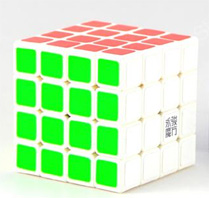4x4 Cube Twisty Puzzle \$14.95 A 4x4 version of Rubik's cube, with a white plastic body (MoYu brand).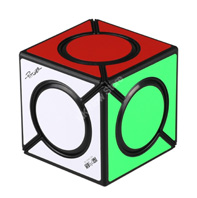Color Spots Twisty Puzzle \$9.95 The corner units turn on this unusual variation on Rubik's-type puzzles.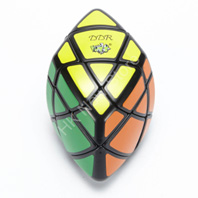Pillow Rhombohedron Twisty Puzzle \$19.95 This puzzle is in the form of a rhombohedron (six faces, each of which is a rhombus), with the faces bulged outward (are pillowed).